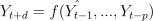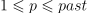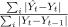# Timeseries#

## Datasets#

mlinsights.timeseries.datasets.artificial_data (dt1, dt2, minutes = 1)

Generates articial data every minutes.

## Experimentation#

mlinsights.timeseries.patterns.find_ts_group_pattern (ttime, values, names, name_subset = None, per = ‘week’, unit = ‘half-hour’, agg = ‘sum’, estimator = None, fLOG = None)

Clusters times series to find similar patterns.

## Manipulation#

mlinsights.timeseries.agg.aggregate_timeseries (df, index = ‘time’, values = ‘y’, unit = ‘half-hour’, agg = ‘sum’, per = None)

Aggregates timeseries assuming the data is in a dataframe.

## Plotting#

mlinsights.timeseries.plotting.plot_week_timeseries (time, value, normalise = True, label = None, h = 0.85, value2 = None, label2 = None, daynames = None, xfmt = ‘%1.0f’, ax = None)

Shows a timeseries dispatched by days as bars.

## Prediction#

The following function builds a regular dataset from a timeseries so that it can be used by machine learning models.

mlinsights.timeseries.selection.build_ts_X_y

The first class defined the template for all timeseries estimators. It deals with a timeseries ine one dimension and additional features.

mlinsights.timeseries.base.BaseTimeSeries (self, past = 1, delay1 = 1, delay2 = 2, use_all_past = False, preprocessing = None)

Base class to build a predictor on timeseries. The class computes one or several predictions at each time, between delay1 and delay2. It computes:with d in [delay1, delay2[ and.

the first predictor is a dummy one: it uses the current value to predict the future.

mlinsights.timeseries.dummies.DummyTimeSeriesRegressor (self, estimator = ‘dummy’, past = 1, delay1 = 1, delay2 = 2, use_all_past = False, preprocessing = None)

Dummy regressor for time series. Use past values as prediction.

The first regressor is an auto-regressor. It can be estimated with any regressor implemented in scikit-learn.

mlinsights.timeseries.ARTimeSeriesRegressor (self, estimator = ‘dummy’, past = 1, delay1 = 1, delay2 = 2, use_all_past = False, preprocessing = None)

Base class to build a regressor on timeseries. The class computes one or several predictions at each time, between delay1 and delay2. It computes:with d in [delay1, delay2[ and.

The library implements one scoring function which compares the prediction to what a dummy predictor would do by using the previous day as a prediction.

mlinsights.timeseries.metrics.ts_mape (expected_y, predicted_y, sample_weight = None)

Computes. It compares the prediction to what a dummy predictor would do by using the previous day as a prediction.